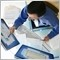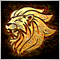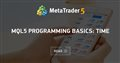# Calculate time left to specific time169

Is there a way to calculate how much time is left from current time to a specific time? Like if I have time string "09:00" and current time is 20:40, how to calculate difference between those times?

That's my code for New York time:

`TimeToStr(TimeLocal() + ((- 4 - Timezone + dstDelta) * 3600), TIME_SECONDS)`

There is 11:43 now and if I have specific time like 09:00 how to calculate how much time left till 09:00?24189

mr-roma: Is there a way to calculate how much time is left from current time to a specific time? Like if I have time string "09:00" and current time is 20:40, how to calculate difference between those times?

Seconds left = specific time - current time. Convert your string to time. If the converted time is in the past, add 24 hours.169

William Roeder:

Seconds left = specific time - current time. Convert your string to time. If the converted time is in the past, add 24 hours.

```   string Time1 = "09:00:00";
string Time2 =  TimeToStr(TimeLocal(), TIME_SECONDS);
WriteText  (del + "SydnayOpens", Time1 - Time2, "Arial", White, 205, 125, "\n", 9, 2, ANCHOR_LEFT_LOWER, FALSE);```

'-' - illegal operation useModerator
13413

You can not subtract string values.

https://www.mql5.com/en/articles/599MQL5 Programming Basics: Time
• www.mql5.com
MQL5 offers a number of simple functions for working with time and you should not find it difficult getting familiar with them. The range of tasks that require use of date and time is quite small. The main tasks are: To perform certain actions at a given point of time (Fig. 1). These may be actions performed at the same time each day or at a...169

Marco vd Heijden:

You can not subtract string values.

https://www.mql5.com/en/articles/599

When I'm converting string to time and subtracting I'm getting some numbers like (-52447) and when I'm converting this numbers back to string with TimeToString() it's not giving me anything24189

mr-roma: I'm getting some numbers like (-52447)

What part of "If the converted time is in the past, add 24 hours," is unclear to you.

mr-roma: when I'm converting this numbers back to string with TimeToString()

What part of “Seconds left =” is unclear to you. You can't convert seconds left to a datetime and then to a string.169

```   datetime SYsT = StrToTime("09:00:00") - (TimeLocal() + (( 11 - Timezone) * 3600));

TimeToStr(SYsT, TIME_SECONDS)```

I finally did it ...

Thanks all